# Resources tagged with: GeoGebra

Filter by: Content type:
Age range:
Challenge level:

There are 143 NRICH Mathematical resources connected to GeoGebra, you may find related items under Physical and Digital Manipulatives.

Broad Topics > Physical and Digital Manipulatives > GeoGebra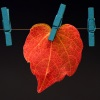### Number Lines in Disguise

##### Age 7 to 14Challenge Level

Some of the numbers have fallen off Becky's number line. Can you figure out what they were?### Robotic Rotations

##### Age 11 to 16Challenge Level

How did the the rotation robot make these patterns?### Polygon Rings

##### Age 11 to 14Challenge Level

Join pentagons together edge to edge. Will they form a ring?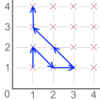### Areas from Vectors

##### Age 11 to 16

Use the applet to explore the area of a parallelogram and how it relates to vectors.### Coordinates of Corners

##### Age 11 to 16

Use the applet to make some squares. What patterns do you notice in the coordinates?### Diminishing Returns

##### Age 11 to 14Challenge Level

How much of the square is coloured blue? How will the pattern continue?##### Age 11 to 16Challenge Level

Draw some quadrilaterals on a 9-point circle and work out the angles. Is there a theorem?### Coded Hundred Square

##### Age 7 to 11Challenge Level

This 100 square jigsaw is written in code. It starts with 1 and ends with 100. Can you build it up?### Pythagoras Proofs

##### Age 14 to 16Challenge Level

Can you make sense of these three proofs of Pythagoras' Theorem?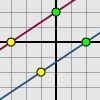### Translating Lines

##### Age 11 to 14Challenge Level

Investigate what happens to the equation of different lines when you translate them. Try to predict what will happen. Explain your findings.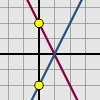### Reflecting Lines

##### Age 11 to 14Challenge Level

Investigate what happens to the equations of different lines when you reflect them in one of the axes. Try to predict what will happen. Explain your findings.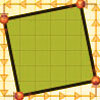### At Right Angles

##### Age 14 to 16Challenge Level

Can you decide whether two lines are perpendicular or not? Can you do this without drawing them?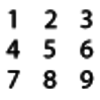### Two-digit Targets

##### Age 5 to 7Challenge Level

You have a set of the digits from 0 â€“ 9. Can you arrange these in the five boxes to make two-digit numbers as close to the targets as possible?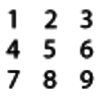### Four-digit Targets

##### Age 7 to 11Challenge Level

You have two sets of the digits 0 â€“ 9. Can you arrange these in the five boxes to make four-digit numbers as close to the target numbers as possible?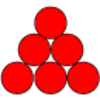### Find the Difference

##### Age 5 to 7Challenge Level

Place the numbers 1 to 6 in the circles so that each number is the difference between the two numbers just below it.### Power Match

##### Age 16 to 18Challenge Level

Can you locate these values on this interactive logarithmic scale?### Which Spinners?

##### Age 14 to 18Challenge Level

Can you work out which spinners were used to generate the frequency charts?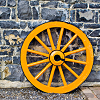### Interactive Spinners

##### Age 11 to 14Challenge Level

This interactivity invites you to make conjectures and explore probabilities of outcomes related to two independent events.### More Twisting and Turning

##### Age 11 to 16Challenge Level

It would be nice to have a strategy for disentangling any tangled ropes...### Sine and Cosine

##### Age 14 to 16Challenge Level

The sine of an angle is equal to the cosine of its complement. Can you explain why and does this rule extend beyond angles of 90 degrees?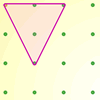### Inside Triangles

##### Age 5 to 7Challenge Level

How many different triangles can you draw on the dotty grid which each have one dot in the middle?### Round and Round and Round

##### Age 11 to 14Challenge Level

Where will the point stop after it has turned through 30 000 degrees? I took out my calculator and typed 30 000 ÷ 360. How did this help?### Where Is the Dot?

##### Age 14 to 16Challenge Level

A dot starts at the point (1,0) and turns anticlockwise. Can you estimate the height of the dot after it has turned through 45 degrees? Can you calculate its height?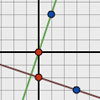### Perpendicular Lines

##### Age 14 to 16Challenge Level

Position the lines so that they are perpendicular to each other. What can you say about the equations of perpendicular lines?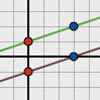### Parallel Lines

##### Age 11 to 14Challenge Level

How does the position of the line affect the equation of the line? What can you say about the equations of parallel lines?### Multiplication Square Jigsaw

##### Age 7 to 11Challenge Level

Can you complete this jigsaw of the multiplication square?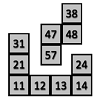### 100 Square Jigsaw

##### Age 5 to 7Challenge Level

Can you complete this jigsaw of the 100 square?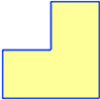### Twice as Big?

##### Age 7 to 11Challenge Level

Investigate how the four L-shapes fit together to make an enlarged L-shape. You could explore this idea with other shapes too.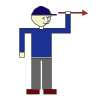### Turning Man

##### Age 5 to 7Challenge Level

Use the interactivity to find out how many quarter turns the man must rotate through to look like each of the pictures.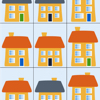### Sort the Street

##### Age 5 to 7Challenge Level

Sort the houses in my street into different groups. Can you do it in any other ways?### More Magic Potting Sheds

##### Age 11 to 14Challenge Level

The number of plants in Mr McGregor's magic potting shed increases overnight. He'd like to put the same number of plants in each of his gardens, planting one garden each day. How can he do it?### Magic Potting Sheds

##### Age 11 to 14Challenge Level

Mr McGregor has a magic potting shed. Overnight, the number of plants in it doubles. He'd like to put the same number of plants in each of three gardens, planting one garden each day. Can he do it?### More Transformations on a Pegboard

##### Age 7 to 11Challenge Level

Use the interactivity to find all the different right-angled triangles you can make by just moving one corner of the starting triangle.### Semi-regular Tessellations

##### Age 11 to 16Challenge Level

Semi-regular tessellations combine two or more different regular polygons to fill the plane. Can you find all the semi-regular tessellations?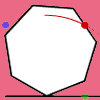### Up and Across

##### Age 11 to 14Challenge Level

Experiment with the interactivity of "rolling" regular polygons, and explore how the different positions of the dot affects its vertical and horizontal movement at each stage.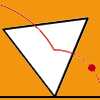### Speeding Up, Slowing Down

##### Age 11 to 14Challenge Level

Experiment with the interactivity of "rolling" regular polygons, and explore how the different positions of the red dot affects its speed at each stage.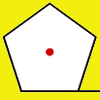### How Far Does it Move?

##### Age 11 to 14Challenge Level

Experiment with the interactivity of "rolling" regular polygons, and explore how the different positions of the red dot affects the distance it travels at each stage.### Mixing More Paints

##### Age 14 to 16Challenge Level

Can you find an efficent way to mix paints in any ratio?### Mixing Paints

##### Age 11 to 14Challenge Level

Can you work out how to produce different shades of pink paint?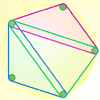### Board Block Challenge

##### Age 7 to 11Challenge Level

Choose the size of your pegboard and the shapes you can make. Can you work out the strategies needed to block your opponent?### Nine-pin Triangles

##### Age 7 to 11Challenge Level

How many different triangles can you make on a circular pegboard that has nine pegs?### Right Angles

##### Age 11 to 14Challenge Level

Can you make a right-angled triangle on this peg-board by joining up three points round the edge?### Subtended Angles

##### Age 11 to 14Challenge Level

What is the relationship between the angle at the centre and the angles at the circumference, for angles which stand on the same arc? Can you prove it?### Triangles in Circles

##### Age 11 to 14Challenge Level

Can you find triangles on a 9-point circle? Can you work out their angles?### Partitioning Revisited

##### Age 11 to 14Challenge Level

We can show that (x + 1)² = x² + 2x + 1 by considering the area of an (x + 1) by (x + 1) square. Show in a similar way that (x + 2)² = x² + 4x + 4### Square Coordinates

##### Age 11 to 14Challenge Level

A tilted square is a square with no horizontal sides. Can you devise a general instruction for the construction of a square when you are given just one of its sides?### Isosceles Triangles

##### Age 11 to 14Challenge Level

Draw some isosceles triangles with an area of $9$cm$^2$ and a vertex at (20,20). If all the vertices must have whole number coordinates, how many is it possible to draw?### Squirty

##### Age 14 to 16Challenge Level

Using a ruler, pencil and compasses only, it is possible to construct a square inside any triangle so that all four vertices touch the sides of the triangle.### Picturing Triangular Numbers

##### Age 11 to 14Challenge Level

Triangular numbers can be represented by a triangular array of squares. What do you notice about the sum of identical triangle numbers?### Guesswork

##### Age 14 to 16Challenge Level

Ask a friend to choose a number between 1 and 63. By identifying which of the six cards contains the number they are thinking of it is easy to tell them what the number is.